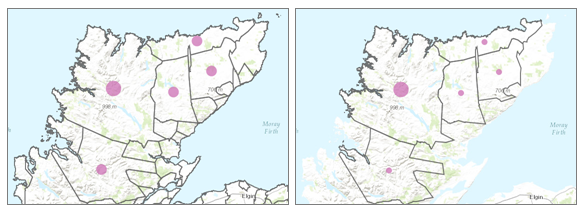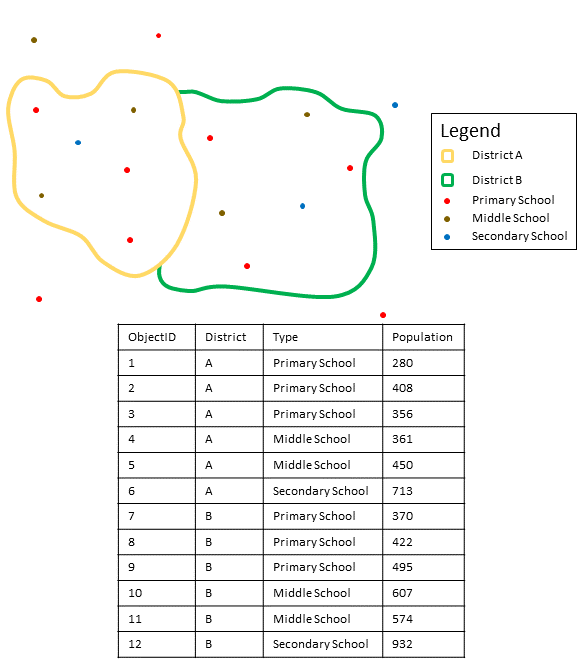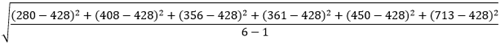# Aggregate Points (Standard Feature Analysis)

## Summary

Uses a layer of point features and a layer of polygon features to determine which points fall within each polygon's area. After determining this point-in-polygon spatial relationship, statistics about all points in the polygon are calculated and assigned to the area.

The most basic statistic is the count of the number of points within the polygon, but you can get other statistics as well. For example, if your points represented coffee shops and each point has a TOTAL_SALES attribute, you can get statistics like the sum of all TOTAL_SALES within the polygon, or the minimum or maximum TOTAL_SALES value, or the standard deviation of all sales within the polygon.

## Illustration## Usage

• Aggregate Points is designed to collect and summarize point features within a set of boundaries. The input parameters must include points to be aggregated and aggregation areas.

• The Keep boundaries with no points box is checked by default. When the box is checked, all of the areas used in the analysis will be included in the result, regardless of the location of points. Areas with no points will be empty and have a count of 0. When the box is unchecked, the areas with no points are completely removed from the result layer. Unchecking the box may have a significant effect on the result areas.The difference in result layers when boundaries with no points are kept (left) or not kept (right)
• The most basic aggregations will calculate a count of the number of points in each boundary. Basic statistics (sum, minimum, maximum, average, and standard deviation) can also be calculated on numerical fields. The statistics will be calculated on each area separately.

• Statistical calculations can also be grouped using a field with categorical values. When statistics are grouped by a field, the statistics are calculated for both the area as a whole and for each group. Both statistics can be viewed in the result layer's pop-up. The overall statistics are given numerically and the grouped statistics are given in chart form within the pop-up. A summary table listing each feature and statistic by group field value will also be created. The Add minority, majority check box will return the groups with the lowest and highest counts and the Add percentages check box will return the percentage of points in the minority and majority groups.

• Point layers are summarized using only the point features within the input boundary. The results will be displayed using graduated symbols.

The figure and table below explain the statistical calculations of a point layer within a hypothetical boundary. The Population field was used to calculate the statistics (Sum, Minimum, Maximum, Average, and Std Deviation) for the layer.Point layers are summarized using only points located within the boundary layer. An example attribute table is displayed above with values to be used in hypothetical statistic calculations.
StatisticResults District A

Sum

``280 + 408 + 356 + 361 + 450 + 713 = 2,568``

Minimum

Minimum of

``[280, 408, 356, 361, 450, 713] = 280``

Maximum

Maximum of

``[280, 408, 356, 361, 450, 713] = 713``

Average

``2,568/6 = 428``

Std Deviation``= 150.79``

## Parameters

### Derived Output

 Label Explanation Data Type Output Layer The output layer. Feature Set Output Group Layer The output table that summarizes the groups from the Group By Field. Record Set

`arcpy.sfa.AggregatePoints(pointLayer, polygonLayer, outputName, {keepBoundariesWithNoPoints}, {summaryFields}, {groupByField}, {minorityMajority}, {percentPoints})`

### Derived Output

 Name Explanation Data Type aggregatedLayer The output layer. Feature Set groupSummary The output table that summarizes the groups from the Group By Field. Record Set

## Licensing information

• Basic: Requires your account in ArcGIS Enterprise to have the Perform Analysis privilege
• Standard: Requires your account in ArcGIS Enterprise to have the Perform Analysis privilege
• Advanced: Requires your account in ArcGIS Enterprise to have the Perform Analysis privilege